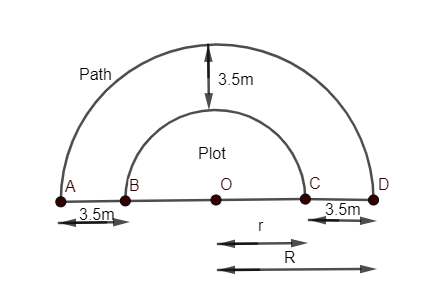Courses
Courses for Kids
Free study material
Free LIVE classes
MoreLIVE
Join Vedantu’s FREE Mastercalss

# A path of width 3.5m runs around a semi-circular grassy plot whose perimeter is 72m. Find the area of the path (Use $\pi =\dfrac{22}{7}$).Verified
363k+ views
Hint: Area of circle is $\pi {{r}^{2}}$ where ‘r’ is the radius of the circle and $\pi =\dfrac{22}{7}$. Perimeter is defined as the continuous line forming the boundary of a closed geometric figure.

Here, we have a path which runs around a semi-circular plot as shown in diagram:-Width of path = 3.5m
Perimeter of plot (semi-circle BC) =72m
As we know that perimeter of any semi-circle can be given as $\pi r+2r$,
where r= Radius of inner semi-circle
$\pi =\dfrac{22}{7}$
Hence, Perimeter of plot as shown in diagram =72
\begin{align} & \pi r+2r=72 \\ & \dfrac{22r}{7}+\dfrac{2r}{1}=72 \\ & \dfrac{22r+14r}{7}=72 \\ \end{align}
or $\dfrac{36r}{7}=72$
So, we get
$r=\dfrac{72\times 7}{36}=14m$.
Now, we can determine the radius of semicircle AD or outer part of the path be OC+CD i.e. r+3.5.
Hence, R=r+3.5m
Where r=14m
So, R=14+3.5=17.5m
As we have to determine area of path which can be given by relation as,
Area of path (ABCD) =Area of outer semi-circle (AD)-Area of inner semi-circle (BC)
We have area of semicircle=$\dfrac{\pi {{r}^{2}}}{2}$
where r is the radius of a semi-circle.
Hence, area of path can be given as
Area of path = $\dfrac{\pi {{R}^{2}}}{2}-\dfrac{\pi {{r}^{2}}}{2}$
where R=17.5m and r=14m and $\pi =\dfrac{22}{7}$.
Area of path = $\dfrac{22}{7}\times \dfrac{1}{2}\times \left( {{R}^{2}}-{{r}^{2}} \right)=\dfrac{11}{7}\left( {{17.5}^{2}}-{{14}^{2}} \right)$
We have algebraic identity $\left( {{a}^{2}}-{{b}^{2}} \right)=\left( a-b \right)\left( a+b \right)$
So, area of path can be rewritten as
Area of path = $\dfrac{11}{7}\left( 17.5-14 \right)\left( 17.5+14 \right)=\dfrac{11}{7}\times 3.5\times 31.5=11\times 0.5\times 31.5$
Area of path$=173.25{{m}^{2}}$

Note: One can go wrong while writing the perimeter of the semi-circle of the plot. He/she may apply the formula ‘$\pi r$’ in place of $\pi r+2r$ which will give the wrong answer. Hence, take care while writing the perimeter of the semi-circle.
Last updated date: 29th Sep 2023
Total views: 363k
Views today: 7.63k